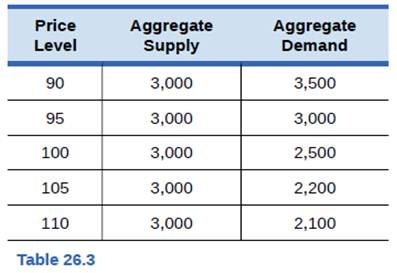Chapter 26, Problem 21P### Principles of Economics 2e

2nd Edition
Steven A. Greenlaw; David Shapiro
ISBN: 9781947172364

#### Solutions

Chapter
Section### Principles of Economics 2e

2nd Edition
Steven A. Greenlaw; David Shapiro
ISBN: 9781947172364
Textbook Problem

# Use Table 26.3 to answer the following questions.a. Sketch an aggregate supply and aggregate demand diagram.b. What is the equilibrium output and price level?C. If aggregate demand shifts right, what is equilibrium output?d. If aggregate demand shifts left, what is equilibrium output?e. In this scenario, would you suggest using aggregate demand to alter the level of output or to control any inflationary increases in the price level?

a)

To determine

Graphical representation of data on AS and AD model.

Explanation

Below diagram is plotted with the given data

b)

To determine

Level of equilibrium output and price.

c)

To determine

Impact on equilibrium level of output due to rightward of AD.

d)

To determine

Impact on equilibrium level of output due to leftward of AD.

e)

To determine

Usage of aggregate demand in altering output.

### Still sussing out bartleby?

Check out a sample textbook solution.

See a sample solution

#### The Solution to Your Study Problems

Bartleby provides explanations to thousands of textbook problems written by our experts, many with advanced degrees!

Get Started

#### Find more solutions based on key concepts